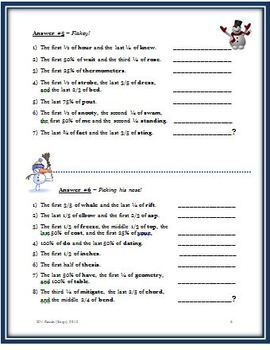Total:
\$0.00# Math Riddles: Reducing Fractions, Identifying Percents and Equivalent Fractions4th - 6th, Homeschool
Subjects
Resource Type
Formats Included
• PDF
Pages
7 pages

#### Also included in

1. This fraction bundle of 13 resources incorporates strategies on how to teach fractions, reduce fractions, work with simple percents, recognize equivalent fractions, find LCM and GCF in addition to adding, subtracting, multiplying and dividing fractions. It contains two projects, four EDITABLE tests,
\$35.00
\$42.00
Save \$7.00
2. This fraction bundle contains six different fraction riddle resources which provide students practice in identifying fractional parts in lowest terms, understanding equivalent fractions as well as identifying basic percents. It is a fun and engaging way to utilize these math concepts while keeping t
\$14.50
\$18.00
Save \$3.50

### Description

This seven page handout has 11 ways to practice recognizing fractional parts, naming equivalent fractions, identifying basic percents (25%, 50%, 75% 100%), and reducing fractions to lowest terms. It is a fun and engaging way to practice math and keep the students actively involved. Specific words are given. The students are instructed to figure out the correct fractional part of each particular word. (Example: The first 50% of WENT would be WE. Notice that WE is also 2/4 or ½ of WENT.) If each fractional part is correctly identified, when the students write the fractional parts on the lines provided, a new word is created. Each group of new words becomes a snow riddle or answer.

It is important that students understand that a fraction and a percent represent the same thing; therefore, many different fractions are represented; however only 25%, 50%, 75% and 100% are introduced.

In the original handout, there are 11 snow word problems. The students answer five riddles and then they are given the answers to six riddles. As a result, they must figure out what the riddle is. Instead of completing all 11 in one day, the puzzles may be divided up and used as a focus activity. Simply give the students one puzzle each day or intersperse them throughout the week or month. Answers are included at the end of the handout.

I also offer different riddles for six months in a 33 page bundle at a discounted price at:

If you prefer to purchase the fraction riddles separately, below are the links. Just click on the title.

Also available on TPT is an 86 page fraction bundle:

Total Pages
7 pages
Included
Teaching Duration
N/A
Report this Resource to TpT
Reported resources will be reviewed by our team. Report this resource to let us know if this resource violates TpT’s content guidelines.